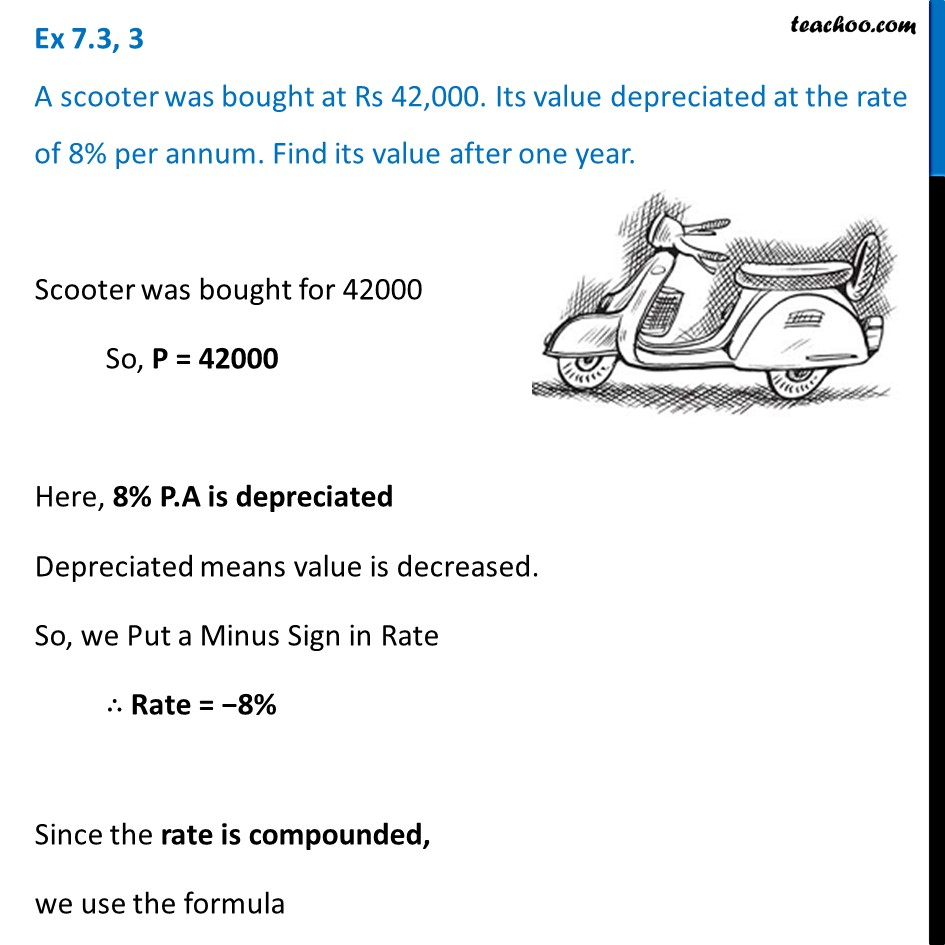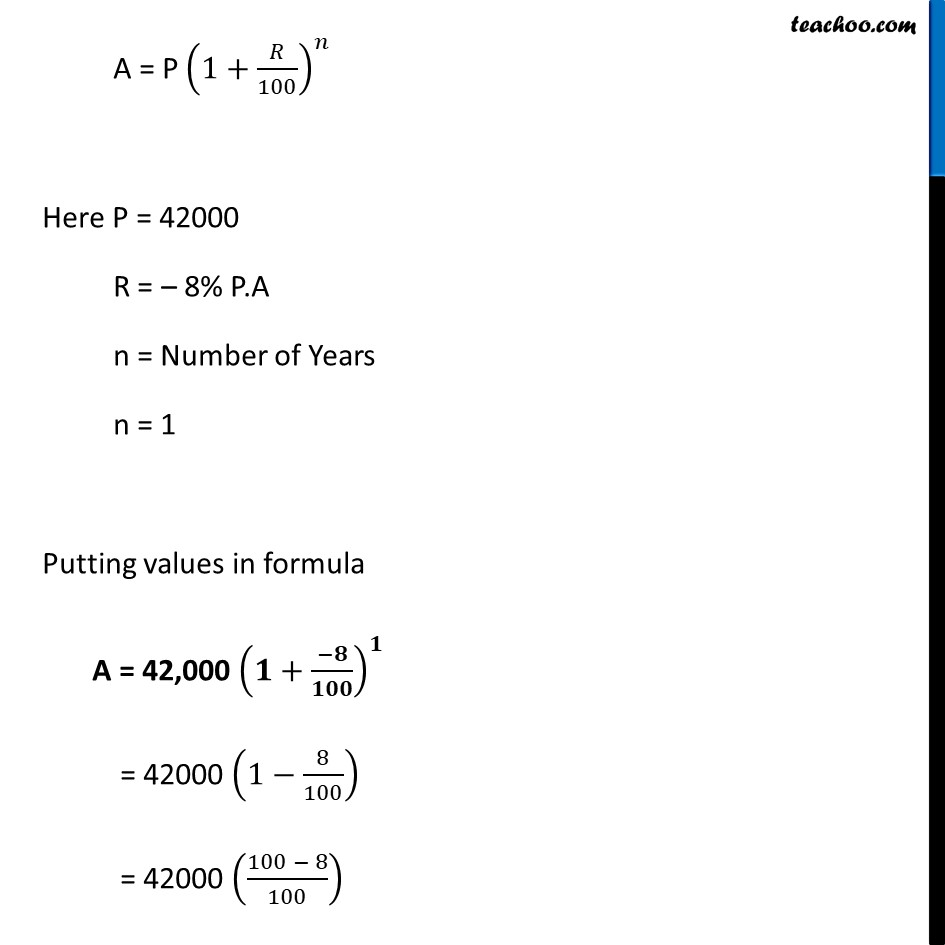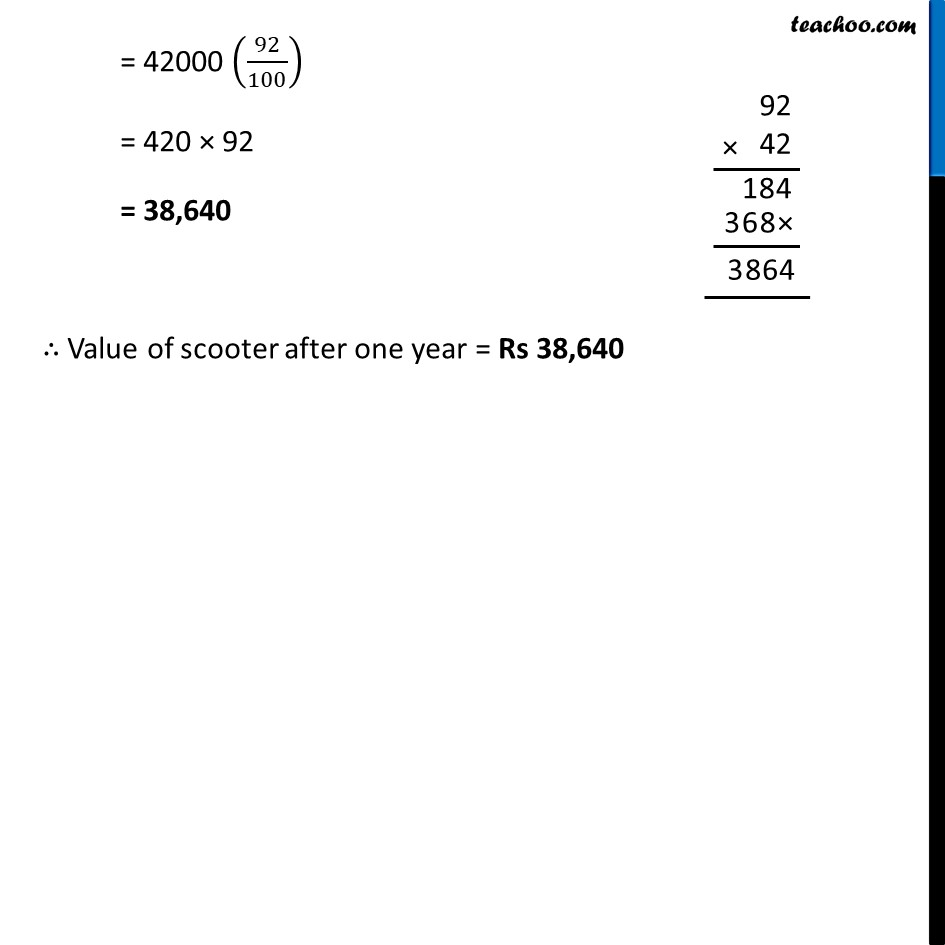Ex 7.3

Chapter 7 Class 8 Comparing Quantities
Serial order wiseLearn in your speed, with individual attention - Teachoo Maths 1-on-1 Class

### Transcript

Ex 7.3, 3 A scooter was bought at Rs 42,000. Its value depreciated at the rate of 8% per annum. Find its value after one year.Scooter was bought for 42000 So, P = 42000 Here, 8% P.A is depreciated Depreciated means value is decreased. So, we Put a Minus Sign in Rate ∴ Rate = −8% Since the rate is compounded, we use the formula A = P (1+𝑅/100)^𝑛 Here P = 42000 R = – 8% P.A n = Number of Years n = 1 Putting values in formula A = 42,000 (𝟏+(−𝟖)/𝟏𝟎𝟎)^𝟏 = 42000 (1−8/100) = 42000 ((100 − 8)/100) = 42000 (92/100) = 420 × 92 = 38,640 ∴ Value of scooter after one year = Rs 38,640